# How Do I Calculate the Area of a Triangle?

Let’s learn how to calculate how much space these super important shapes take up.Author
Taylor HartleyExpert Reviewer

Published: August 24, 2023# How Do I Calculate the Area of a Triangle?

Let’s learn how to calculate how much space these super important shapes take up.Author
Taylor HartleyExpert Reviewer

Published: August 24, 2023# How Do I Calculate the Area of a Triangle?

Let’s learn how to calculate how much space these super important shapes take up.Author
Taylor HartleyExpert Reviewer

Published: August 24, 2023Key takeaways

• The area of a triangle is how much surface space the triangle takes up – Don’t confuse it with the perimeter, which is the measure of the triangle’s exterior sides of these sides’ lengths.
• Calculate a triangle’s area using base and height – Multiply the base of the triangle by its height, then cut that number in half (by dividing it by 2 or multiplying it by ½).
• Use the area formula – If you have the base and area, use the formula to solve for height, and vice versa if you know the area and height.

Triangles are some of the most important shapes you’ll encounter in the real world. They’re everywhere, from a slice of pizza or a tortilla chip to the great pyramids of Egypt! Triangles are also some of the most important shapes to use when constructing homes, skyscrapers, and more, so it’s a good idea to know how to calculate exactly how much space they take up.

Today, we’ll learn how to calculate the area of a triangle, or how much space a triangle takes up. Once you know how to find a triangle’s area, you’ll be well on your way to understanding how they work in the real world!

## First, what is a triangle?A triangle is a polygon with three sides and three vertices (or the points where each of these sides meet). These three-sided polygons also have three interior angles, which all add up to a sum of 180 degrees.

Fun fact: the triangle is the polygon with the least number of sides, angles, and vertices possible.

## The different types of trianglesThere are different types of triangles, classified either by the measure of their sides or the measure of their angles. Let’s explore the different types of triangles.

### Scalene triangleA scalene triangle is a triangle with three different side lengths, and three angles with different measurements. In other words, none of the sides of a scalene triangle are equal to one another, and none of its three angles have the same measurement.

### Equilateral triangleAn equilateral triangle is the exact opposite of a scalene triangle. Each side of an equilateral triangle is the same length, and each of its three angles have the same measurement. Everything regarding an equilateral triangle is, as its name suggests, equal!

### Isosceles triangleAn isosceles triangle is a triangle that has two equal sides, and two equal angles. The two equal angles are formed directly opposite of the two equal sides. This leaves one side, and one angle opposite to it that aren’t equal.

### Right triangleA right triangle is a triangle with one right angle, or an angle with a measurement of 90°. The other two angles in this triangle usually measure 45°, but they can be any size, so long as together, they add up to 180°. As long as one angle within the triangle is 90°, it’s considered a right triangle.

### Obtuse triangleAn obtuse triangle is a triangle with one interior angle with a value greater than 90°. In an obtuse triangle, since one angle is already greater than 90°, the sum of the other two angles must be less than 90°. Remember, the sum of a triangle’s three angles must equal 180°.

### Acute triangleAn acute triangle is a triangle in which all three angles are less than 90°. The sum of these angles will still add up to 180°, but each of these angles will only ever have values less than 90°.

## So, what is the area of a triangle?The area of a triangle refers to the total amount of space included within the three lines of the shape. To calculate the area, we have to first identify the size of the triangle’s base and height. Because the base and height will always vary by triangle, you’ll encounter different triangles with vastly different areas.

That’s why it’s important for you to master the basic formula for calculating the area of a triangle. But be careful: don’t confuse the area of a triangle with the perimeter. Confused on the difference between the two? Let’s take a look.

### What’s the difference between area and perimeter?The area of a triangle refers to the amount of space enclosed within the three-sided polygon. The perimeter of a triangle is the sum of the measurements of each side.

## The basic formula for finding the area of a triangleThe formula for calculating the area of a triangle is pretty basic, and relies solely on the measurements of the base and height. If you’re able to find both of these values, you can easily calculate the area of a triangle. The formula below shows how to use these values to find the area of a triangle: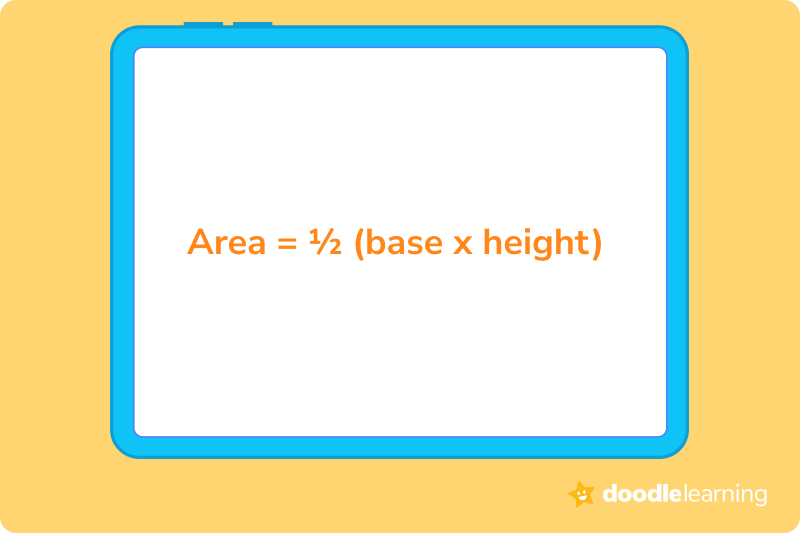Another way to write this formula is: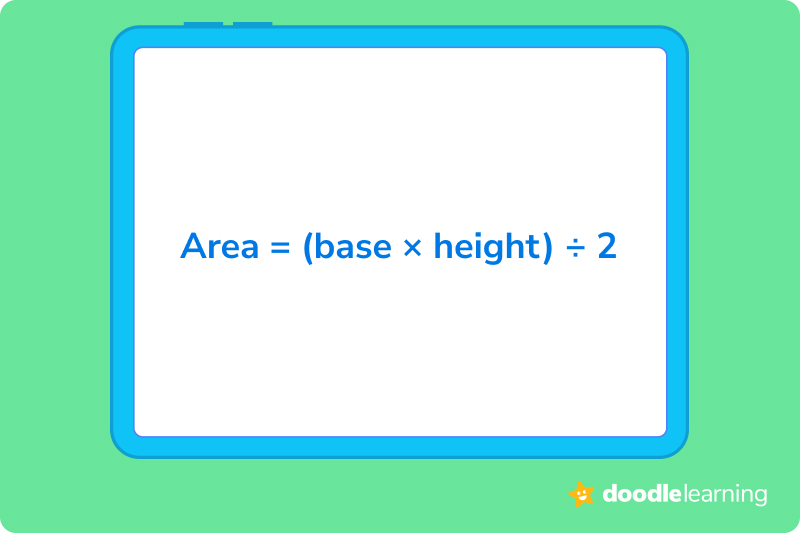Choose whichever formula is easier for you! In the first, you are multiplying by ½ and in the second, you are dividing by 2. These will give you the same result either way, so choose the formula that makes the most sense to you.

## Other formulas for other trianglesAs you continue your journey in studying mathematics, there may be other formulas you’ll need to learn and practice for calculating the area of a triangle. Lucky for you, you’ll mainly encounter these formulas in higher level math courses, where the properties of the triangle become a bit more complicated.

For now, let’s stick to the fundamentals and make sure we master calculating the area of more basic triangles.

## Let’s practice together!### 1. Imagine a triangle with a base of 6 units and a height of 4 units. Calculate its area.Let’s go step-by-step to solve this problem:

1. Write down the formula you know.

Area = (base x height) ÷ 2

2. Plug in the values.

Area = (6 x 4) ÷ 2

3. Multiply.

Area = 24 ÷ 2

4. Divide.

Area = 12 square units

### 2. The base of a triangle is 10 units and the height is 8 units. Can you find its area?Okay, let’s do it together!

1. Write down the formula.

Area = (base x height) ÷ 2

2. Plug in the values.

Area = (10 x 8) ÷ 2

3. Multiply.

Area = 80 ÷ 2

4. Divide.

Area = 40 square units

### 3. Picture a triangle with a base of 7 units and a height of 3 units. Then, calculate the area.One more time to make sure we’ve got it!

1. Write down the formula.

Area = (base x height) ÷ 2

2. Plug in the values.

Area = (7 x 3) ÷ 2

3. Multiply.

Area = 21 ÷ 2

4. Divide.

Area = 10.5 square units

Ready to give it a go?

Now that we’ve run through a few practice problems together, it’s time for you to tackle a few on your own.

Feel free to scroll back to earlier in our article if you struggle to remember the formula for finding the area, or if you aren’t sure exactly how to apply it. And don’t get discouraged if things get difficult. Area can be a difficult thing to understand, so just keep practicing until you feel more comfortable.

## Practice problems

The area is 36 square units.

The area is 42 square units.

The triangle has an area of 75 square units.

The area of this triangle is 20 square units.

## Parent Guide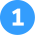The area is 36 square units

How did we get here?

1. First, let’s identify how our numbers fit into our area formula: Area = ½ (8 x 9)
2. Now, we can multiply 8 and 9 together: 8 x 9 = 72
3. Next, we need to multiply our product from step 2 (72) by ½ : 72 x ½ = 36
4. The area of the triangle is equal to 36 square units.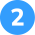The area is 42 square units

How did we get here?

1. Write our formula down: Area = ½ (12 x 7)
2. Now we multiply: 12 x 7 = 84
3. Now we multiply 84 by ½ (in other words, divide 84 by 2).
4. 84 x ½ = 42
5. The area of the triangle is 42 square units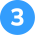The triangle has an area of 75 square units.

How did we get here?

1. Write out our formula: Area = ½ (15 x 10)
2. Multiply 15 by 10: 15 x 10 = 150
3. Now multiply 150 by ½ : 150 x ½ = 75
4. Area = 75 square units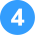The area of this triangle is 20 square units.

How did we get here?

1. Write out the formula: Area = ½ (5 x 8)
2. Multiply the factors within the parentheses: (5 x 8) = 40
3. Multiply 40 by ½ : 40 x ½  = 20
4. Area = 20 square units

## FAQs about math strategies for kidsWe understand that diving into new information can sometimes be overwhelming, and questions often arise. That’s why we’ve meticulously crafted these FAQs, based on real questions from students and parents. We’ve got you covered!

The area of a triangle is the space enclosed within the three sides of any basic triangular shape. Calculating the area tells us just how much space these polygons take up, which is helpful when trying to understand how to apply them to world problems and other real world situations.

The area of a triangle relies on knowing the measurements for both the base of the triangle, and its height. Once you know both of these values, you can multiply them together, and then multiply that product by ½ . The result of that equation provides you with the area. As you learn higher levels of math, you’ll begin to apply other formulas for finding the areas of more advanced kinds of triangles.

We can use the same formula we use to calculate the area to find both the base and height. However, we need to already know the value of the area and the value of either the base or the height if we’re going to the counterpart. For example, if the area and base are both known, this means we can solve for the height. If the area and height are known, we can solve for the base. All we need to do is use our original area formula.

Triangles are a fundamental part of how we construct our environment, both physically and virtually. They are an exceptionally strong kind of shape, making them the perfect foundation for constructing anything from skyscrapers to bridges. They’re perhaps the most important unit of building.## Related Posts

Title 1

Title 2

Title 3

Lesson credits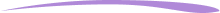Taylor Hartley

Taylor Hartley is an author and an English teacher based in Charlotte, North Carolina. When she's not writing, you can find her on the rowing machine or lost in a good novel.

Jill Padfield has 7 years of experience teaching high school mathematics, ranging from Alegra 1 to AP Calculas. She is currently working as a Business Analyst, working to improve services for Veterans while earning a masters degree in business administration.

Taylor Hartley

Taylor Hartley is an author and an English teacher based in Charlotte, North Carolina. When she's not writing, you can find her on the rowing machine or lost in a good novel.

Jill Padfield has 7 years of experience teaching high school mathematics, ranging from Alegra 1 to AP Calculas. She is currently working as a Business Analyst, working to improve services for Veterans while earning a masters degree in business administration.# Are you a parent, teacher or student?

Are you a parent or teacher?

## Hi there!

Book a chat with our team